# Sequences and Series

## Homework Statement

Find the sum of the products of every pair of the first 'n' natural numbers.

## Homework Equations

sigma n^2 = n(n+1)(2n+1)/6

## The Attempt at a Solution

S=1.2 + 1.3 + 1.4 .....+ 2.3 + 2.4 ........n-1(n)
i cant figure out how to proceed ..

Dick
Homework Helper
Think about expanding the expression (1+2+...+n)*(1+2+...+n) like it was a polynomial.

(1+2+...n)^2 = (1.1 +2.2 ......n.n) + (1.2+1.3 ...1.n .....n-1.n)

Dick
Homework Helper
You have a formula for summing the squares 1^2+2^2+...+n^2. You've probably got a formula for summing 1+2+..+n as well. Use it.

AGNuke
Gold Member
That's a good question and very conceptual. Try to break your problem into a general summation which can be expressed by a variable.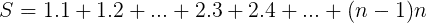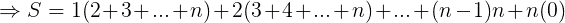Don't you worry about n(0), that thing is created to be zero, as you'll see in next step.

We can take the numbers outside the brackets as r, whose value varies from r=1,2,...,(n-1),n.

Now about the summation of numbers inside the bracket. You can take out their sum by AP. Since the first term of any bracket is (r+1) and the last term is always n, the number of terms can be figured as (n-r), since you're not counting first r natural number.

Therefore, our general term can be written as: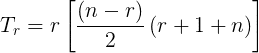NOTE: Try considering r=n. It is a huge relief that Tn=0, or else we would have to manually subtract it in the end. Always consider manually checking last term in the general term, they may give you a problem.

Now fearlessly take summation of our series.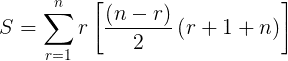Now open it and you'll get the following: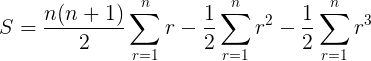Simple equation in summation of r, r2 and r3

That's a good question and very conceptual. Try to break your problem into a general summation which can be expressed by a variable.Don't you worry about n(0), that thing is created to be zero, as you'll see in next step.

We can take the numbers outside the brackets as r, whose value varies from r=1,2,...,(n-1),n.

Now about the summation of numbers inside the bracket. You can take out their sum by AP. Since the first term of any bracket is (r+1) and the last term is always n, the number of terms can be figured as (n-r), since you're not counting first r natural number.

Therefore, our general term can be written as:NOTE: Try considering r=n. It is a huge relief that Tn=0, or else we would have to manually subtract it in the end. Always consider manually checking last term in the general term, they may give you a problem.

Now fearlessly take summation of our series.Now open it and you'll get the following:Simple equation in summation of r, r2 and r3
summing up your equation , i got
n[n+1/2]^2 [ (3n-2)(n-1) / 12 ]
will you check if this right . . . .

AGNuke
Gold Member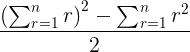This is a better solution, but I was afraid of it since I was not fully sure whether it will work or not, but I guess in the end, my hunch was right!Its simple enough. Let's see.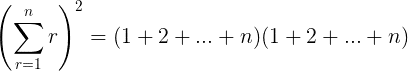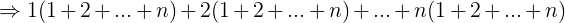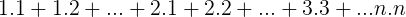As you can see, after multiplying, some squares are obtained, which we remove by Ʃr2.

Further more, You can see every non-square term will repeat two time, so we divide the remaining part by 2 and here we are.This is a better solution, but I was afraid of it since I was not fully sure whether it will work or not, but I guess in the end, my hunch was right!Its simple enough. Let's see.As you can see, after multiplying, some squares are obtained, which we remove by Ʃr2.

Further more, You can see every non-square term will repeat two time, so we divide the remaining part by 2 and here we are.
Thanx AGNuke , that was some real help !

AGNuke
Gold Member
Welcome!